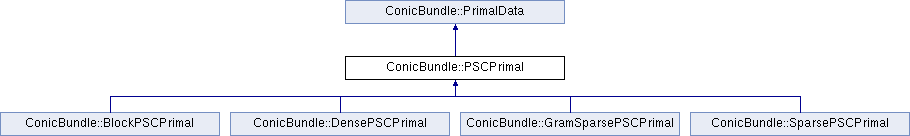ConicBundle
ConicBundle::PSCPrimal Class Referenceabstract

PSCPrimal is the corresponding positive semidefinite object for PSCOracle like PrimalMatrix for a MatrixFunctionOracle. More...

`#include <PSCPrimal.hxx>`

Inheritance diagram for ConicBundle::PSCPrimal:## Public Member Functions

virtual PrimalDataclone_primal_data () const =0
returns a newly generated identical Object

virtual int assign_Gram_matrix (const CH_Matrix_Classes::Matrix &P)=0
intialize the primal corresponding to the (positive semidefinite) Gram matrix P*P^T

virtual int aggregate_primal_data (const PrimalData &pd, double factor=1.)=0

virtual int aggregate_Gram_matrix (const CH_Matrix_Classes::Matrix &P, double factor=1.)=0

virtual int scale_primal_data (double factor)=0
multiply/scale *this with a nonnegative factor

virtual int primal_ip (CH_Matrix_Classes::Real &value, const SparseCoeffmatMatrix &A, CH_Matrix_Classes::Integer column) const =0
if compatible evaluate value=ip(*this,A.column[i])

## Detailed Description

PSCPrimal is the corresponding positive semidefinite object for PSCOracle like PrimalMatrix for a MatrixFunctionOracle.

It allows the system to aggregate eigenvector information obtained in successive evaluations of the maximum eigenvalue function in PSCOralce to a positive semidefinite matrix so that in the Lagrangian relaxation view the primal optimal semidefinite matrix can be approximated, see abstract positive semidefinite cone oracle.

ConicBundle::PSCModel does rely on primal information. It also does not care about whether and in which form primal information is represented. This is all hidden in the Minorant information obtained via PSCOracle::generate_minorant(). If provided, the primal information can be retrieved from the model by the routines PSCModel::get_approximate_primal() and PSCModel::get_center_primal() or via the corresponding routines in the interface.

The documentation for this class was generated from the following file: